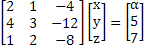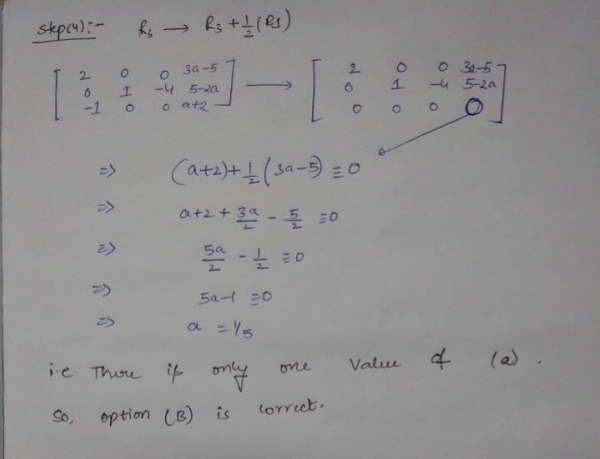Related Articles

# GATE | GATE-CS-2003 | Question 41

• Last Updated : 08 Jan, 2019

Consider the following system of linear equationsNotice that the second and the third columns of the coefficient matrix are linearly dependent. For how many values of a, does this system of equations have infinitely many solutions?
(A) 0
(B) 1
(C) 2
(D) infinitely many

Explanation: